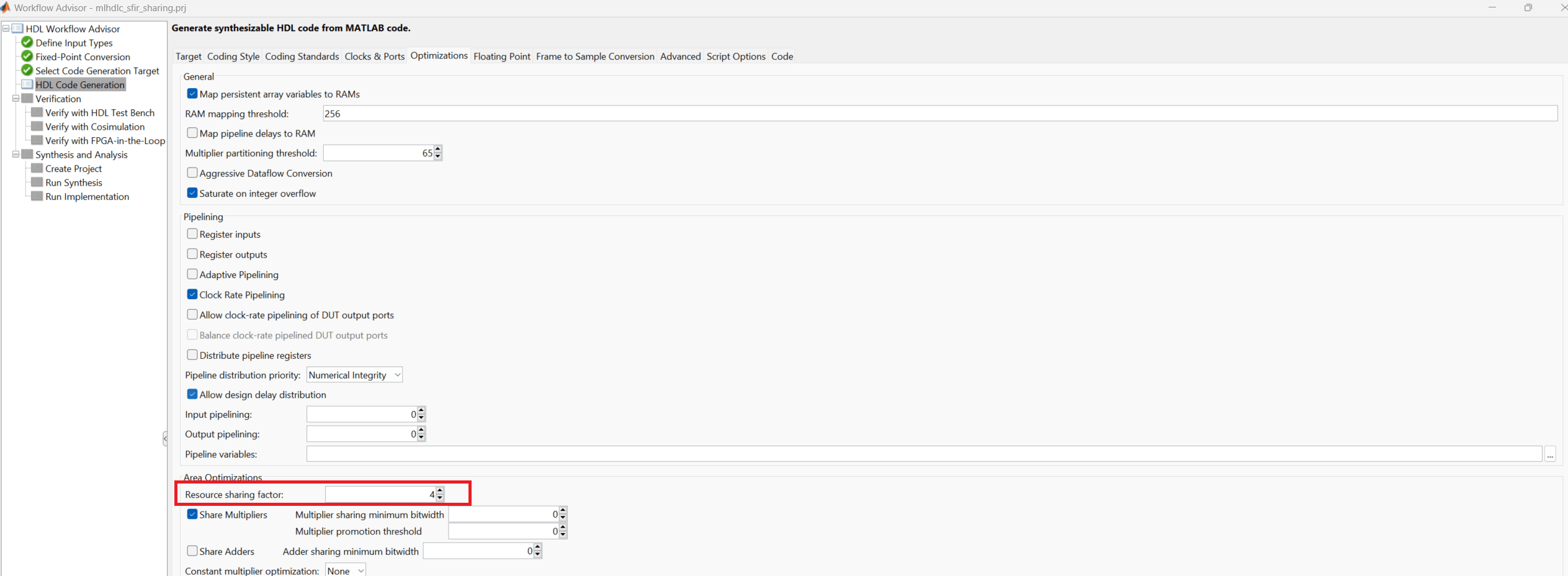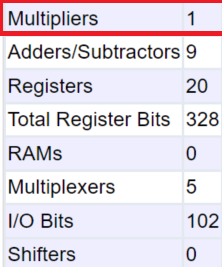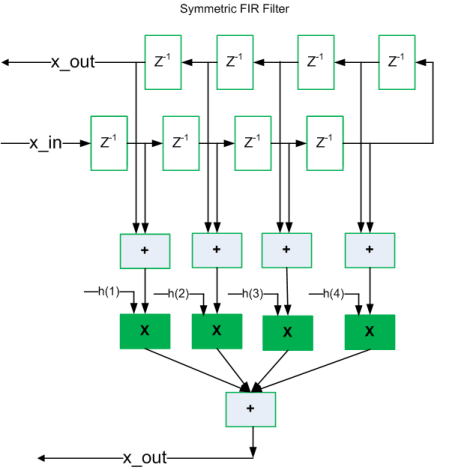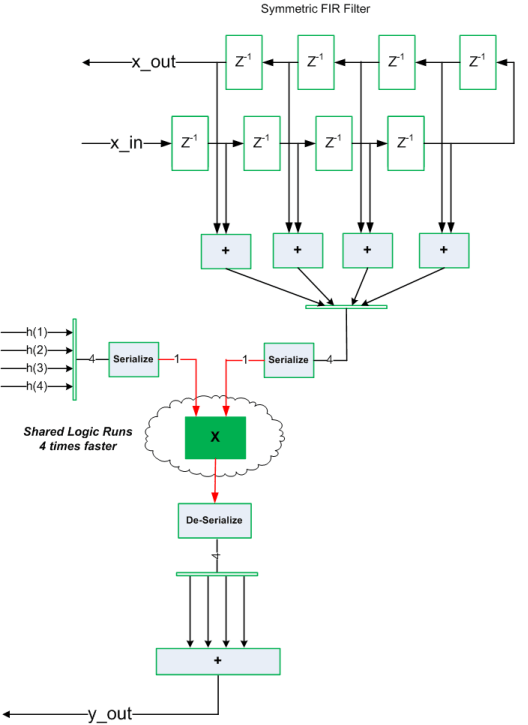# Resource Sharing of Multipliers to Reduce Area

This example shows how to use the resource sharing optimization in HDL Coder™. This optimization identifies functionally equivalent multiplier operations in MATLAB® code and shares them in order to optimize design area. You have control over the number of multipliers to be shared in the design.

### Introduction

Resource sharing is a design-wide optimization supported by HDL Coder™ for implementing area-efficient hardware.

This optimization enables users to share hardware resources by mapping `N` functionally-equivalent MATLAB operators, in this case multipliers, to a single operator.

The user specifies `N` using the `Resource Sharing Factor` option in the optimization panel.

Consider the following example model of a symmetric FIR filter. It contains 4 product blocks that are functionally equivalent and which are mapped to 4 multipliers in hardware. The Resource Utilization Report shows the number of multipliers inferred from the design.

In this example you run fixed-point conversion on the MATLAB design `mlhdlc_sharing` followed by HDL Coder. This prerequisite step normalizes all the multipliers used in the fixed-point code. You input proposed-type settings during this fixed-point conversion phase.

### MATLAB Design

The MATLAB code used in the example is a simple symmetric FIR filter written in MATLAB and also has a testbench that exercises the filter.

```design_name = 'mlhdlc_sharing'; testbench_name = 'mlhdlc_sharing_tb'; ```

Look at the MATLAB design.

```type(design_name); ```
```%%%%%%%%%%%%%%%%%%%%%%%%%%%%%%%%%%%%%%%%%%%%%%%%%%%%%%%%%%%%%%%%%%%%%%%%%% % MATLAB design: Symmetric FIR Filter % % Key Design pattern covered in this example: % (1) Filter states represented using the persistent variables % (2) Filter coefficients passed in as parameters %%%%%%%%%%%%%%%%%%%%%%%%%%%%%%%%%%%%%%%%%%%%%%%%%%%%%%%%%%%%%%%%%%%%%%%%%% % Copyright 2011-2015 The MathWorks, Inc. %#codegen function [y_out, x_out] = mlhdlc_sharing(x_in, h) % Symmetric FIR Filter persistent ud1 ud2 ud3 ud4 ud5 ud6 ud7 ud8; if isempty(ud1) ud1 = 0; ud2 = 0; ud3 = 0; ud4 = 0; ud5 = 0; ud6 = 0; ud7 = 0; ud8 = 0; end x_out = ud8; a1 = ud1 + ud8; a2 = ud2 + ud7; a3 = ud3 + ud6; a4 = ud4 + ud5; % filtered output y_out = (h(1) * a1 + h(2) * a2) + (h(3) * a3 + h(4) * a4); % update the delay line ud8 = ud7; ud7 = ud6; ud6 = ud5; ud5 = ud4; ud4 = ud3; ud3 = ud2; ud2 = ud1; ud1 = x_in; end ```
```type(testbench_name); ```
```%%%%%%%%%%%%%%%%%%%%%%%%%%%%%%%%%%%%%%%%%%%%%%%%%%%%%%%%%%%%%%%%%%%%%%%%%% % MATLAB test bench for the FIR filter %%%%%%%%%%%%%%%%%%%%%%%%%%%%%%%%%%%%%%%%%%%%%%%%%%%%%%%%%%%%%%%%%%%%%%%%%% % Copyright 2011-2015 The MathWorks, Inc. clear mlhdlc_sharing; % input signal with noise x_in = cos(3.*pi.*(0:0.001:2).*(1+(0:0.001:2).*75)).'; len = length(x_in); y_out = zeros(1,len); x_out = zeros(1,len); % Define a regular MATLAB constant array: % % filter coefficients h = [-0.1339 -0.0838 0.2026 0.4064]; for ii=1:len data = x_in(ii); % call to the design 'mlhdlc_sfir' that is targeted for hardware [y_out(ii), x_out(ii)] = mlhdlc_sharing(data, h); end figure('Name', [mfilename, '_plot']); plot(1:len,y_out); ```

### Create a New HDL Coder Project

Run the following command to create a new project:

```coder -hdlcoder -new mlhdlc_sfir_sharing ```

Next, add the file `mlhdlc_sharing.m` to the project as the MATLAB Function and `mlhdlc_sharing_tb.m` as the MATLAB Test Bench.

Refer to Get Started with MATLAB to HDL Workflow for a more complete tutorial on creating and populating MATLAB HDL Coder projects.

### Realize an N-to-1 Mapping of Multipliers

Turn on the resource sharing optimization by setting the 'Resource Sharing Factor' to a positive integer value.

This parameter specifies `N` in the N-to-1 hardware mapping. Choose a value of N > 1.### Examine the Resource Report

There are 4 multiplication operators in this example design. Generating HDL with a `SharingFactor` of 4 will result in only one multiplier in the generated code.### Sharing Architecture

The following figure shows how the algorithm is implemented in hardware when we synthesize the generated code without turning on the sharing optimization.The following figure shows the sharing architecture automatically implemented by HDL Coder when the sharing optimization option is turned on.

The inputs to the shared multiplier are time-multiplexed at a faster rate (in this case 4x faster and denoted in red). The outputs are then routed to the respective consumers at a slower rate (in green).### Run Fixed-Point Conversion and HDL Code Generation

Launch the Workflow Advisor and right-click the Code Generation step. Choose the option Run to selected task to run all the steps from the beginning through the HDL code generation.

The detailed example Fixed-Point Type Conversion and Derived Ranges provides a tutorial for updating the type proposal settings during fixed-point conversion.

Note that to share multipliers of different word-length, in the Optimization -> Resource Sharing tab of HDL Configuration Parameters, specify the Multiplier promotion threshold.

### Run Synthesis and Examine Synthesis Results

Synthesize the generated code from the design with this optimization turned off, then with it turned on, and examine the area numbers in the resource report.